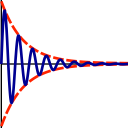Subsections

## deselect.sn_ratio### Synopsis

Deselect spins with signal to noise ratio higher or lower than the given ratio.

### Defaults

deselect.sn_ratio(ratio=10.0, operation=`<', all_sn=False)

### Keyword arguments

ratio: The signal to noise ratio to compare to.

operation: The comparison operation by which to deselect the spins.

all_sn: A flag specifying if all the signal to noise ratios per spin should match the comparison operator, of if just a single comparison match is enough.

### Description

The comparison operation is the method which to deselect spins according to: operation(sn_ratio, ratio).

The possible operations are: `<':strictly less than, `<=':less than or equal, `>':strictly greater than, `>=':greater than or equal, `==':equal, `!=':not equal.

The `all_sn' flag default is False, meaning that if any of the spin's signal to noise levels evaluates to True in the comparison, the spin is deselected.

### Prompt examples

To deselect all spins with a signal to noise ratio lower than 10.0:

```[numbers=none]
relax> deselect.sn_ratio(ratio=10.0, operation='<')
```

```[numbers=none]
relax> deselect.sn_ratio(ratio=10.0, operation='<', all_sn=True)
```

The relax user manual (PDF), created 2020-08-26.